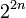# Difference between revisions of "Quiz:Degrees of irreducible representations"

## Find the feasible or infeasible degrees of irreducible representations

1 Which of the following is not a possibility for the multiset of the degrees of irreducible representations of a finite group over a splitting field of characteristic zero (such as the complex numbers)?

 1,1,1,1,2 1,1,1,1,3 1,1,1,1,4 None of the above, i.e., they are all possibilities for the multiset of degrees of irreducible representations.

2 Which of the following is not a possibility for the multiset of the degrees of irreducible representations of a finite group over a splitting field of characteristic zero (such as the complex numbers)?

 1,1,2,3,3 1,1,1,2,2,2,3 1,1,1,1,2,2,2,2,2 1,1,1,1,1,1,1,2,2,3 1,1,1,1,1,1,1,1,2,2,2,2

3 Which of the following is not a possibility for the multiset of the degrees of irreducible representations of a finite group over a splitting field of characteristic zero (such as the complex numbers)?

 1,1 1,1,2 1,1,1,3 1,1,1,1,4 None of the above, i.e., they are all possibilities

1 Which of the following statements is false in general about the degrees of irreducible representations of a finite group over a splitting field of characteristic zero?

 The degree of any irreducible representation divides the index of any abelian subgroup in the group. The degree of any irreducible representation is bounded by, but need not divide, the index of any abelian subgroup in the group. The degree of any irreducible representation divides the index of any abelian normal subgroup in the group.

2 What is the largest possible value of the maximum degree of irreducible representation for a group of order 24 over a splitting field of characteristic zero (such as the complex numbers)?

 2 3 4 6 8

3 What is the largest possible value of the maximum degree of irreducible representation for a group of order$2^{2n + 1}$ over a splitting field of characteristic zero (such as the field of complex numbers) where$n$ is a positive integer?

 2$2^n$$2^{n + 1}$$2^{2n - 1}$$2^{2n}$$2^{2n + 1}$